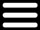# Walleye Weight Calculator

Below is the Walleye weight formula that the calculator is based on.

The formula to calculate the weight for a Walleye that is 20 inches long and has a girth of 12 inches is as follows. Walleye have a shape factor of 750.

WEIGHT = LENGTH x GIRTH2 / SHAPE FACTOR

WEIGHT= 20 x (122) / 750
WEIGHT= 20 x 144 / 750
WEIGHT= 2880 / 750
WEIGHT = 3.84 pounds (This would be a very fat Walleye)

 Length Girth Fish Type Calculated Weight . Walleye Pike/Musky (1-120 inches) (1-100 inches) . (pounds)